# Thorn Fractals

After seeing Paul Bourke’s page about Thorn Fractals I had to have a play and add them into Visions Of Chaos.

Sample C source code is available on Paul’s page and the inner formula comes down to CX and CY parameters in the main iteration loop for each pixel;

a = ir;
b = ii;
ir = a / cos(b) + cr;
ii = b / sin(a) + ci;

Here are some sample images using the above formula. The CX and XY in this case can be anywhere between -10 and +10.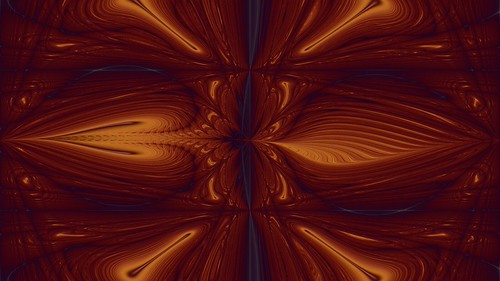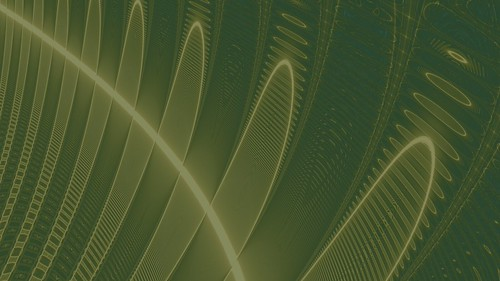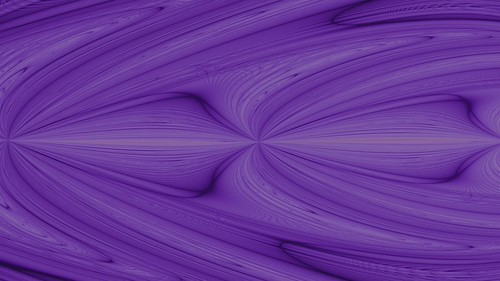I also experimented with changing the formulas and discovered the following three variations.

Variation 1.

ir:=a/cos(b)*sin(a)+cx;
ii:=b/sin(a)*cos(b)+cy;

For this variation the CX and CY should stay within the range of -1 and +1. Values outside this range will lead to very noisy images.Variation 2.

ir:=a/cos(b)*sin(b)+cx;
ii:=b/sin(a)*cos(a)+cy;

Again CX and CY should stay within the range of -1 and +1.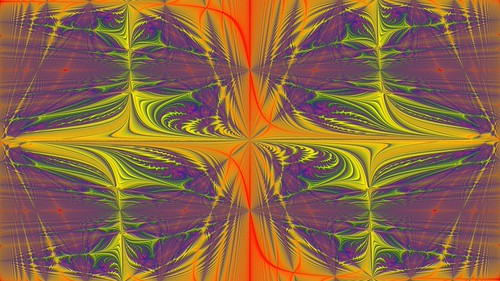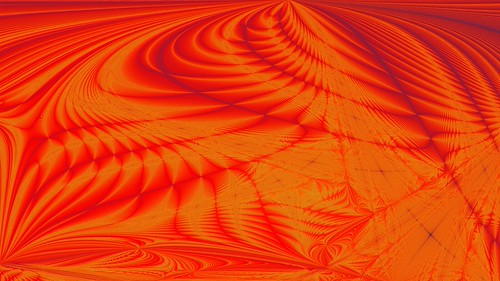Variation 3.

ir:=a/sin(cos(b)*sin(b))+cx;
ii:=b/cos(sin(a)*cos(a))+cy;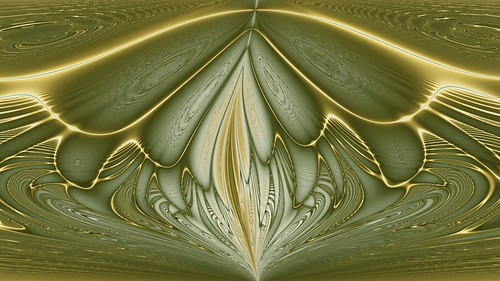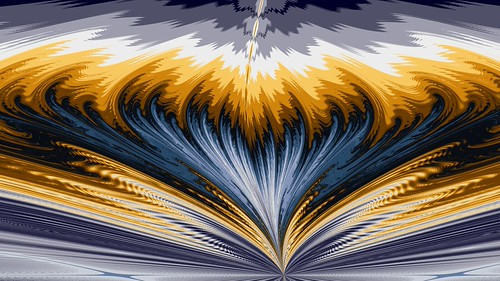When rendering these sorts of fractal images you will get lots of aliasing, noise and moire effects. These can be overcome by supersampling (rendering the image to 10 or 20 times the actual size and downsampling). Most of the images in my sample gallery used at least 10 times supersampling, so every pixel is actually the average of 100 sub-pixel calculations. Even at 20 times supersampling there are still visible areas that show aliasing in some renders.

Dane Vandeputte (mentioned on Paul’s page) uses Contrast Limited Adaptive Histogram Equalization to bring out some really nice details in these sort of fractals. I will have to try adding CLAHE to Visions Of Chaos in the future.

Jason.